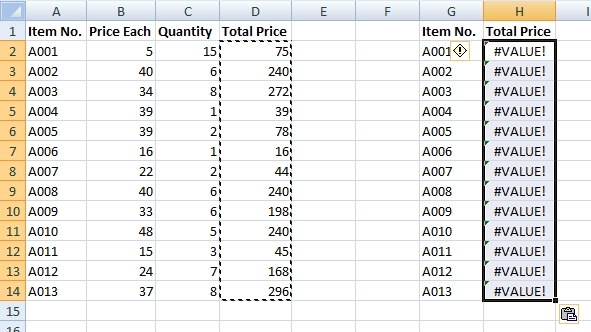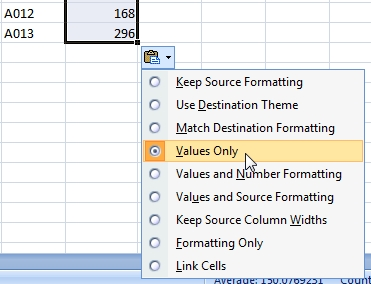# How to change the formula to value

There are many reasons for replacing formula with its result.

For example 1: If you delete cells belonging to the formula, the result of the formula goes wrong. For preservation the result, you have to change the formula to the value. After that other changes in cells will have no effect.

For example 2: When you copy a cell with a formula, the source cells are changing and therefore the result is changing too. However, this behavior is not always appropriate. You can solve it by adding letter \$ into the formula or by changing the formula to a value.

## Example

Let’s see how to do that.In the column D – Total Price is formula =B2*C2. We want to copy Total Price from column D to column H.

Here is the result if we do a simple copy. As you see, there is error value #VALUE. It is because of the formula change to =F2*G2.To fix this, you have to click on the button Paste Options and select Values Only. Excel will convert the result of the formula to the static value.1.Thanx. I use this trick for data mining in big complex tables with a lot of formulas included.

2.How can I remove formula from the cell? I want keep value only.

3.DO IT